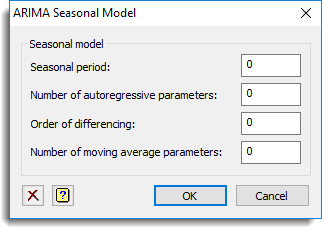1. Home
2. ARIMA Seasonal Model

# ARIMA Seasonal Model

You can extend the basic ARIMA model to include a simple seasonal part, comprising components for seasonal autoregression, seasonal differencing, and seasonal moving-average.

The Seasonal Model is specified in the same way as the basic ARIMA model, with an additional parameter denoting the seasonal period. For example, when analysing data collected at monthly intervals you may wish to specify a seasonal period of 12, thus allowing for annual effects in the data.## Seasonal period

This specifies the number of observations in the seasonal period. For example if this was days in a year the number would be 365, and if quarters in a year it would be 4. If this is zero, no seasonal model will be applied to the data.

## Number of autoregressive parameters

This specifies the number of autoregressive parameters that are used to model the seasonal effects. If this is zero, no autoregressive parameters will be used.

## Order of differencing

This gives the order of differencing applied to the seasonal effects. If this is zero, the seasonal effects will not be differenced.

## Number of moving average parameters

This specifies the number of moving average parameters that are used to model the seasonal effects. If this is zero, no moving average parameters will be used.